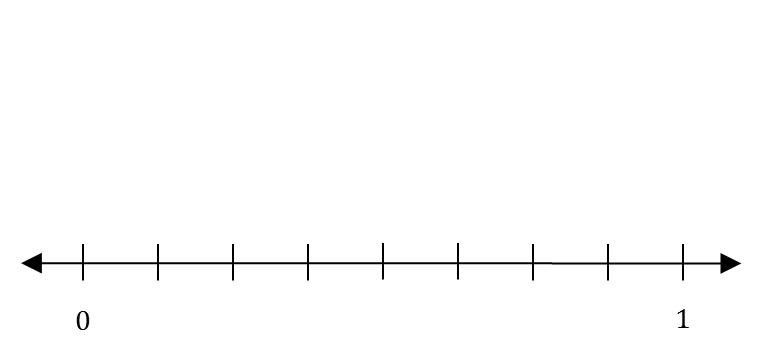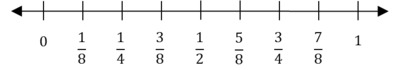# Fractions on a Line Plot

Alignments to Content Standards: 5.NF.A.1 5.MD.B.2

You and your partner will need fraction cards made from this set:1. Label the line-plot below with $\frac18$'s. Cut out and divide the cards evenly between the two players, laying them face-down. Each partner will choose one of their face-down cards and turn it over. The team will then add their fractions together. For each turn, each team will record their sum on the line plot.Each team should have 12 data points marked on their line plot.

2. Look at the line plot. Which values came up the most? Which values did not come up?
3. The tick marks on the number line correspond to eighths. Which of the eighths will never come up as a sum of two of these cards? Why?
4. You want to improve the game so that it is possible for two fractions to sum to $\frac{7}{8}$. Name one fraction card that you could add to the deck and explain why your new card would now make it possible to have $\frac{7}{8}$ as a sum of two cards.

## IM Commentary

The purpose of this task is for students to add unit fractions with unlike denominators and solve addition and subtraction problems involving fractions that have more than one possible solution (5.NF.1). The reason that students are asked to record their results on a line plot is that they need a way to systematically record their sums and to help them reason about the possible sums they can make with the cards. While this task does not ask students to record measurement data (as described in 5.MD.2), it is a good introductory activity for working with line plots with non-whole numbers.

This activity would be best during the early months of 5th grade when students are gaining familiarity with adding and subtracting unlike fractions and strengthening their understanding of equivalency on the number line. The three fractions introduced can all be represented in eighths, thus making this task ideal to build fluency with fraction operations where one denominator is a factor of the other. If the teacher would like to add an extra level of challenge, he or she can label the number line with fractions as shown below, which would require students to find equivalent fractions for some of the sums.The task also pushes students to engage in MP.3, Construct Viable Arguments and Critique the Reasoning of Others. Students will use their data and their reasoning to make conjectures about which values could not come up. Though their experimental data will likely point them in the right direction, they will need to create an organized system to prove that certain values cannot come up. If this task is discussed in a whole group after individuals or pairs have had an opportunity to think about the follow-up questions, there should be opportunities for students to show different ways of constructing their arguments.

This task is designed to be used in class. If students become comfortable with this type of task, it could be used as a center in centers-based learning. Though the activity is for pairs, the questions posed after students create the line plot would be good to discuss as a whole group after students have had a chance to discuss them in pairs.

A printable copy of the cards is attached to this task.

## Attached Resources

• Fraction Cards Master
• ## Solution

1. Before discussing the individual solutions, it is helpful to think about the possible outcomes in this task.

Card 1 Card 2 Sum
$\frac18$ $\frac18$ $\frac28$
$\frac18$ $\frac14$ $\frac38$
$\frac14$ $\frac18$ $\frac38$
$\frac18$ $\frac12$ $\frac58$
$\frac12$ $\frac18$ $\frac58$
$\frac14$ $\frac14$ $\frac48$
$\frac14$ $\frac12$ $\frac68$
$\frac12$ $\frac14$ $\frac68$
$\frac12$ $\frac12$ $\frac88$

There should be 12 “x”s marked on the line plot and these “x”s should only be above the values $\frac{2}{8}, \frac{3}{8}, \frac{4}{8}. \frac{5}{8}, \frac{6}{8}$ or 1. One possible example is shown below:2. Answers may vary, but in the example line plot above, $\frac{3}{8}$, came up most frequently and 0, $\frac18$, $\frac68$, and $\frac78$ did not come up. Fifth grade students are not expected to work on concepts of probability. The purpose of this question is to prime students to think about which answers cannot come up at all rather than to explain why some possible sums are more likely than others.

3. The values that cannot come up are 0, $\frac{1}{8}$ and $\frac{7}{8}$ because none of the combinations of the cards will sum to these values, as we saw above.

Students might also note that 0 and $\frac{1}{8}$ are both less than or equal to one of the addends. Since 5th graders have not yet worked with negative numbers, they could not start with $\frac{1}{8}$ and add a non-zero value and end with a sum less than or equal to $\frac{1}{8}$ (and none of the cards have negative numbers).

There are 3 possibilities for new cards, $\frac34$, $\frac58$, or $\frac38$:

\begin{align} \frac18+\frac34 &=\frac78\\ \frac14+\frac58 &=\frac78\\ \frac12+\frac38 &=\frac78\\ \end{align}
Students should be able to show how they found the sums above.